Ch 4. Fundamental Laws (Integral Anal.) Multimedia Engineering Fluids ConservationMass LinearMomentum Moment ofMomentum ConservationEnergy
 Chapter 1. Basics 2. Fluid Statics 3. Kinematics 4. Laws (Integral) 5. Laws (Diff.) 6. Modeling/Similitude 7. Inviscid 8. Viscous 9. External Flow 10. Open-Channel Appendix Basic Math Units Basic Equations Water/Air Tables Sections Search eBooks Dynamics Fluids Math Mechanics Statics Thermodynamics Author(s): Chean Chin Ngo Kurt Gramoll ©Kurt GramollFLUID MECHANICS - THEORY

In this section, the definitions of a system and control volume are stated. The principle of conservation of mass will be introduced. The conservation of mass equation in integral form will be presented for both fixed and moving control volumes.

Definitions: System and Control Volume

A system is a fixed quantity of matter, and no mass is allowed to cross the system boundary. A control volume is a space of interest where mass can cross the boundary. The boundary of the control volume is called the control surface.

Conservation of Mass Equation:
Fixed, Nondeforming Control Volume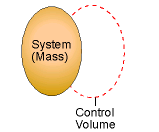Conservation of Mass
for a Control Volume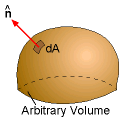Outward Normal Vector

As the name implies, the principle of conservation of mass states that mass is conserved for a system. Applying the conservation of mass principle for a fixed and nondeforming control volume: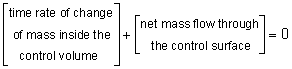The statement above can be easily expressed in mathematical form to yield the conservation of mass equation for a control volume as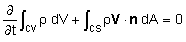where n is the outward unit normal vector, which is used to describe the orientation of the control surface. Hence the net mass flow out of the control volume is considered positive.

For steady flows, the total mass in the control volume will not vary with time, and the first term vanishes.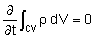The second term in the conservation of mass equation represents the net mass flow through the control surface. For control volume with a finite number of faces, it is sometimes convenient to write it in discrete form as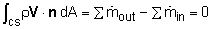where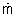is the mass flow rate. This can also be written as,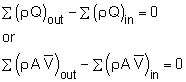where ρ is the density of the fluid, Q is the volumetric flow rate and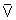is the average velocity perpendicular to the cross sectional area A.

If there is just one inlet and one outlet and the density does not change, which is common, this relationship is simply,

A1V1 = A2V2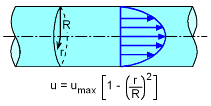Pipe Flow with
Parabolic Velocity Profile

The average velocity can be obtained by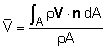Consider flow in a pipe as shown in the figure. The average velocity can be obtained by integrating the parabolic velocity profile to yield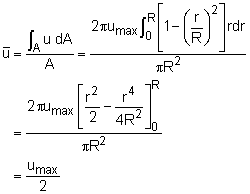Conservation of Mass Equation:
Moving, Nondeforming Control Volume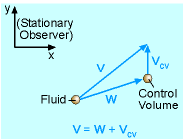Relative Velocity

The conservation of mass equation for a moving and nondeforming control volume is the same as described above, but the velocity V is replaced with the relative velocity W.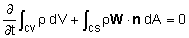From a stationary observer point of view, the control volume moves at a velocity Vcv, while the fluid moves at a velocity of V. W is the relative velocity of the fluid with respect to the moving control volume. These velocities are related by

V = W + Vcv

Practice Homework and Test problems now available in the 'Eng Fluids' mobile app
Includes over 250 problems with complete detailed solutions.
Available now at the Google Play Store and Apple App Store.